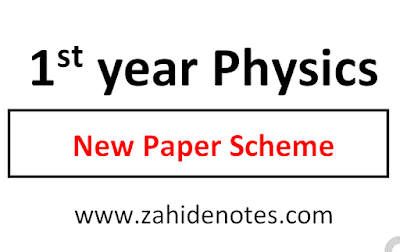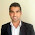The paper Scheme of physics for 1st year 2021 for the Punjab board is now published on Zahid Notes. The students can have a look at the scheme and follow it in their studies. Zahid Notes already provides all and every this that is helpful for the students.So, on this website, you will see the guess papers, study schemes, notes, paper patterns, and all other staff that is relevant to your studies.

# 1st year physics paper scheme 2021

You should see:
1. 2nd year scheme 2021 in pdf
2. 1st year paper patterns
3. 9th class physics paper scheme 2021
4. 10th class paper scheme 2021

The scheme of physics for first year is now latest and fresh. This is for the year 2021. You will get latest content at Zahid Notes. The paper scheme of physics first year is given below. Download 1st year physics solved numericals in pdf.

Pairing schemes are usually the division of marks and paper questions for every chapter. So, I have given the scheme in both image for and the text for to copy and share through SMS.

This scheme is valide for all Punjab boards like Lahore board, multan board, Sargodha board, DG Khan board, Faisalabad board, etc.

You may want to: download 1st year physics complete notes in pdf

## Pairing scheme of physics 1st year class 11

 Chapter No. MCQs Short Questions Long Questions 1 2 4 NIL 2 2 3 1 3 2 4 1 4 1 3 1 5 2 3 1 6 1 1 1 7 1 3 1 8 2 3 1 9 1 3 1 10 1 2 1 11 2 4 1

Q#2(Ch# 1, 2, 3, 6)       Q#3(Ch# 4, 5, 7, 8)    Q#4(Ch# 9, 10, 11)

Q#5(Ch# 2, 3)      Q#6(Ch# 4, 8)       Q#7(Ch# 5, 6)

Q#8(Ch# 7, 11) Q#9(Ch# 9, 10)

Question No. 1 MCQs = 17 (1 from chapter 4, 6, 7, 9, 10 each and 2 mcqs from chapter 1, 2, 3, 5, 8, 11 each)

#### Short questions

Question No. 2 (8/12)
Chapter 1 = 4
Chapter 2 = 3
Chapter 3 = 4
Chapter 6 = 1

Question No. 3 (8/12)
Chapter 4 = 3
Chapter 5 = 3
Chapter 7 = 3
Chapter 8 = 3

Question No. 4 (6/9)
Chapter 9 = 3
Chapter 10 = 2
Chapter 11 = 4

#### Long questions (Attempt ANY THREE questions from FIVE Questions)

Questions No. 5
Chapter 2 = part A
Chapter 3 = Part B

Questions No. 6
Chapter 4 = part A
Chapter 8 = Part B

Questions No. 7
Chapter 5 = part A
Chapter 6 = Part B

Questions No. 8
Chapter 7 = part A
Chapter 11 = Part B

Questions No. 9
Chapter 9 = part A
Chapter 10 = Part B

You may also like:
1st year notes pdf
Pairing scheme 2021 1st year physicsReviewed by Saif Ullah ZahidonMay 31, 2021 Rating: 5

1.2.3.4.5.6.Very very thanks sir rrr really🙂🙂🥰

7.Sir ap tashreh b upload kr skty chapters ki?

8.excuse me sir? my physics professor said that chapter 2 has been excluded from extensive questions section this year (2021).Are you sure that this scheme is a totally updated version?

1.i feel the same too cause all the other sites have different schemes

9.Kis kis ko selena Gomez Nazar I 😂😂😂

10.Numericals knsy chap sy any ha

11.guys are you sure this pairing scheme is right
I prepared the long question according to it
if this is not authentic then tell me my future depends on it

12.Is this scheme true??

1.13.Is this scheme true

14.Sindh board ki scheme kahan h?

15.Sir yh tu bta dy ky numerical kis ch ky part ma ana h

1.2.Bro B part hota hi numerical ka a

3.Pakii bat ha first part me nii aty naww numerical

16.17.18.Thank you but sir numericals kis chapter sa ha

19.Assalam o alaikum sir plz can u inform us about numerical scheme??

1.Sir g numerical kn sy chapter k krny gain according sheme